# Using a Genetic Algorithm for Traveling Salesman Problem in Python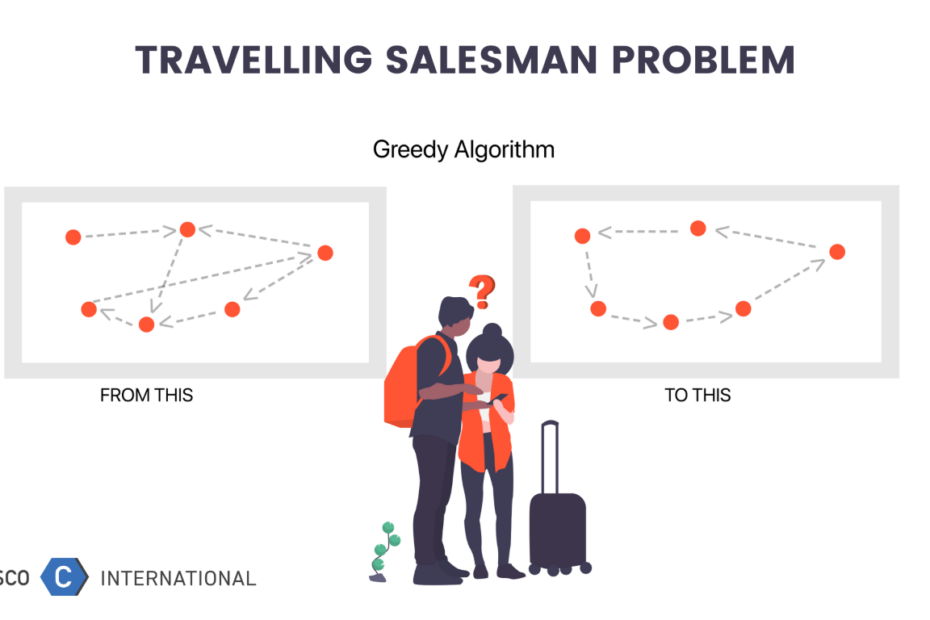#### What are heuristics algorithms?

While solving large scale linear/integer problems, it becomes extensively difficult to solve or even reach a feasible solution within the prescribed practical duration. To mitigate such issues, it is a common practice in optimization community to resort various heuristics algorithms and reach a feasible solution which may or may not be an optimum solution. Some of the heuristic algorithms are listed below:

–         Greedy Search

–         Tabu Search

–         Depth first Search

–         Genetic Algorithm

–         Particle Swarm Optimization

–         Bee Colony Optimization

Heuristics algorithms are meant to find an approximate solution as the search algorithm does not traverse through all the possible solution.

Genetic Algorithm (GA):

In this article, we will understand the functions involved in genetic algorithm and try to implement it for a simple Traveling Salesman Problem using python.

GA is a search-based algorithm inspired by Charles Darwin’s theory of natural evolution. GA follows the notion of natural selection. The process of natural selection starts with the selection of fittest individuals from a population. They produce offspring which inherit the characteristics of the parents and will be added to the next generation. If parents have better fitness, their offspring will be better than parents and have a better chance at surviving. This process keeps on iterating and at the end, a generation with the fittest individuals will be found.

We can use this notion for search-based algorithm. We can formally state this process in as following phases:

1.      Initial population

2.      Fitness Function

3.      Selection

4.      Cross-over

5.      Mutation

The basic flow of GA can be represented by this diagram: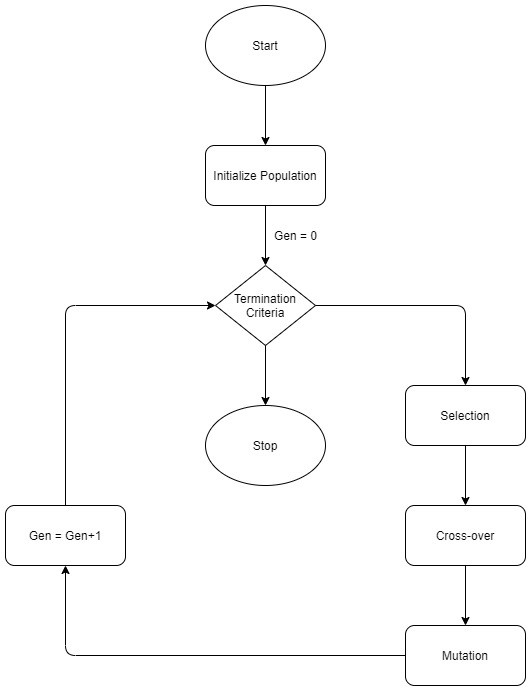#### Traveling Salesman Problem (TSP) using GA:

As TSP is a well-known problem, we will just summarize in a single line as: Given a list of cities and the distances between each pair of cities, what is the shortest possible route that visits each city and returns to the origin city?

Keeping this in mind there are two rules that needs to be considered:

–         Each location just be visited only once.

–         The route should return to the location from where it started.

Considering the phases of GA, we now apply it in the context of GA:

–         Gene – A location represented as (x,) co-ordinate.

–         Individual (chromosome) – a single route satisfying the above stated conditions.

–         Population – Collection of feasible individuals (routes).

–         Parents – Two individuals selected to create future individuals.

–         Mating pool – Collection of parents for next generations.

–         Fitness function – An objective function which gives the information of how good the individual is for further selection.

–         Mutation – a method to bring in a variation by randomly swapping two cities in a route.

–         Elitism – term which means to carry the best individuals to the next generation.

Considering the above steps, we will look at the code for the same:

–         Gene – Creating a class of location which has x and y as its attributes. We also include a distance calculator function inside the class using a simple Pythagorean theorem. We can also use haversine function for better result. Here we just keep things simple.

.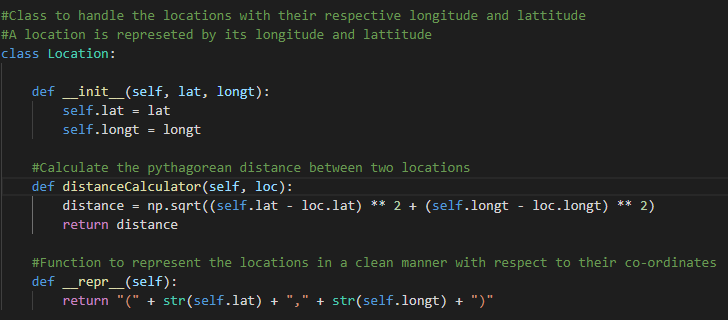–         Fitness Function – We want to minimize the route distance. Here we consider an inverse of the distance, hence a larger fitness function value is desirable.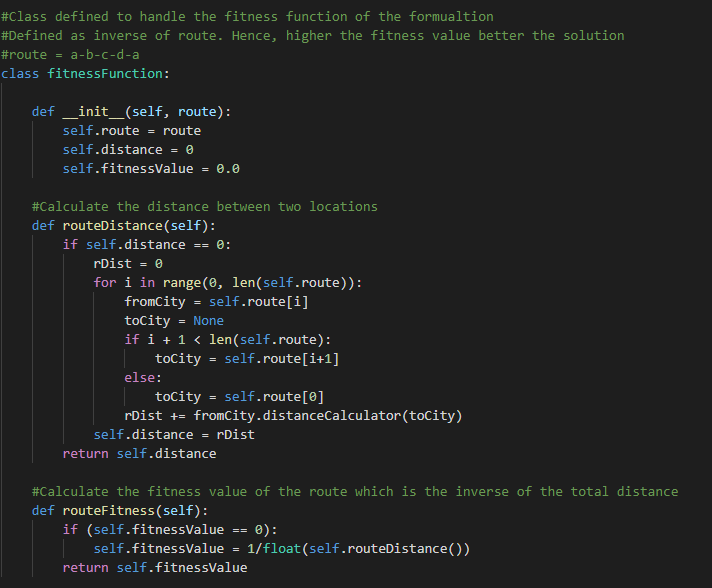–         Create Population: We need to first initialize the first generation for that we create two functions, first which will create a random route and second to create a population sample. These functions will be utilized only to create the initial population as subsequent population will be generated by mutation and cross-over.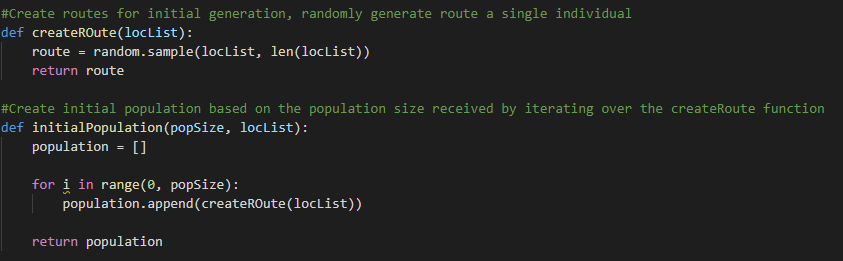–         Mating Pool Selection – Determining the fitness, we need to rank the fitness values so that we have an ordered list of the same. Now we need to implement a selection procedure. There are multiple ways to select the parents for future generation. The most common practices are roulette wheel selection or tournament selection. In this code, we implement the roulette wheel selection idea which is assigning each individual probability of selection based on their fitness value. Another design feature to consider is the use of elitism. With elitism, the best performing individuals from the population will automatically carry over to the next generation, ensuring that the most successful individuals persist. We do this in two steps, first we rank the routes and then apply the roulette wheel selection and introduce the elitism scheme in the selection procedure.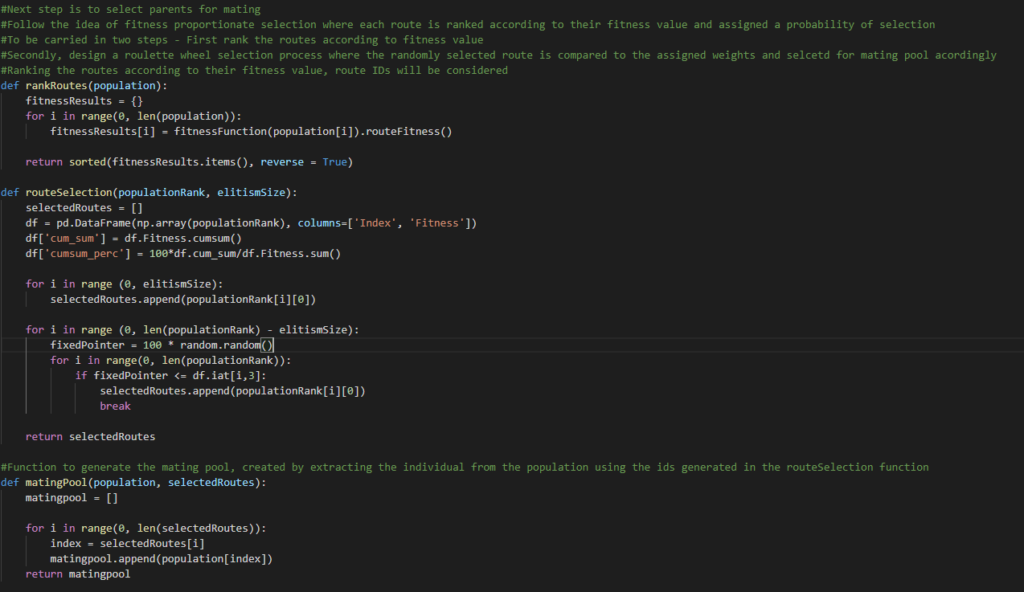–         Cross-Over – While performing the cross over operation we consider two-point cross over. The following image depicts a binary string crossover. However, TSP is unique as we need one location to appear just once. The code will depict the procedure adopted. We take a randomly selected subset of the parent string and fill the remainder of the route with the genes of the second parent to avoid any duplicates.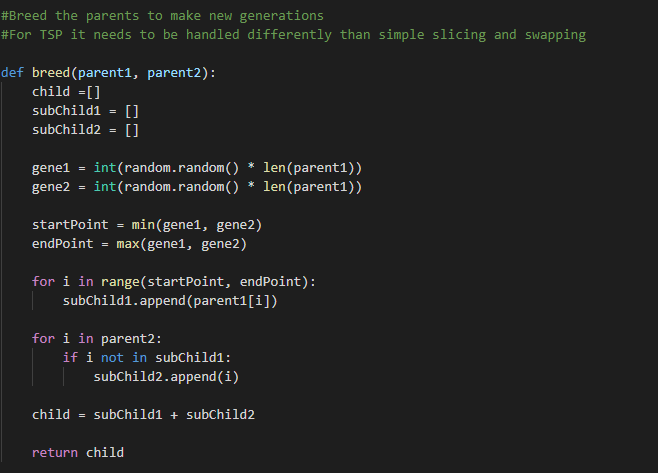Next, we generalize this operation to create the population for the next generation..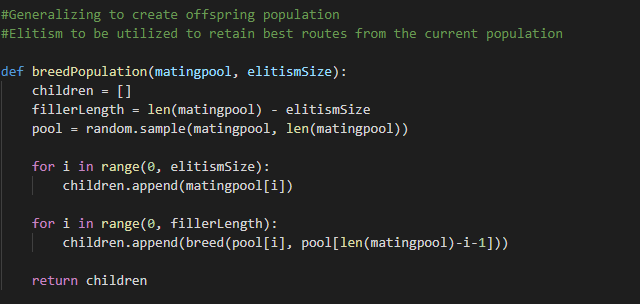–         Mutation – This operation is an important procedure in GA, so that the algorithm can avoid local convergence and introduce novel routes in the population to explore other parts of the solution space. In TSP, we use swapped mutation technique that is we swap two cities in the route.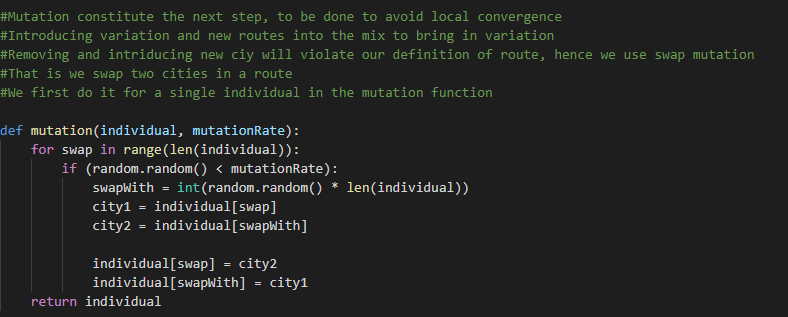Next, we generalize it to the population.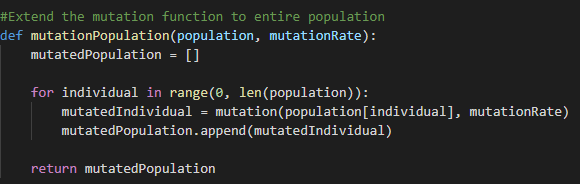–         Create Generations – Having all the above operations in place, we now move on to create a function that implements them to create the generations. We follow the steps:

o  Rank routes

o  Selection

o  Mating Pool

o  Cross-over population

o  Mutate population.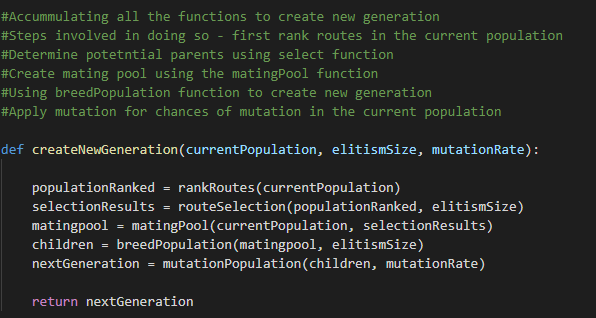–         Implementing GA – Now, the only task remaining is to create initial population and run the GA pieces that we have put together until now. We create a function to implement GA with the required parameters, i.e., generations, mutation rate, population size, initial population, elitism size.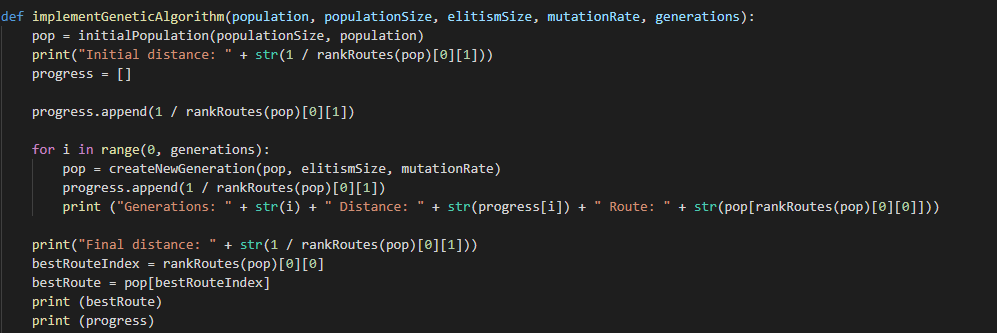–         Running the algorithm – Now, we just need to call the function with are desired parameters.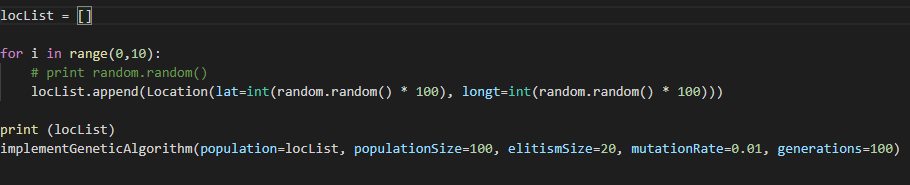#### Conclusion:

GA is not the answer to all problems, the solution can be problem specific. But we can be sure that arriving to a solution using this algorithm is fast which may or may not be optimal. Based on the problem in hand, we can implement the desired heuristic algorithms.

#### References:

Genetic Algorithms in search, optimization and machine learning – David E. Goldberg